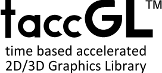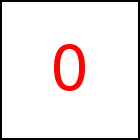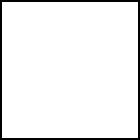# Expressions - taccGL™ Tutorial

## Relative Coordinates

Using JavaScript you can assign a transition to a JavaScript variable. Later on you can access information about this transition. For example

 var step=taccgl.actor("testimg"); step.to (step.x, step.y+500, 0).start(); RUN

This defines a transition named step but does not yet use start (1st line). Then it uses attributes step.x and step.y of step. These give the position of the underlying HTML element (testimg in this case) in pixels from the documents top left.

The parameters for to are expressions step.x and step.y+500. This means the destination x-coordinate is identical to the x coordinate of the HTML element itself. So the object moves just top-down, leaving x-coordinate constant. For y it uses step.y+500. This means the object moves 500 pixels down.

Note: you always need to finish the JavaScript var using a semicolon ";" before you can access the new transition and its attributes.

## More Complex Examples

A very common use of expressions is to specify relative coordinates as shown in the example and section above.

 var step=taccgl.a("testimg").paint(); step.to (step.x, step.y+step.h+30 , 0).start().cont().start(); RUN

This example uses step.h which gives the elements height. It moves the image 30 pixels below its original bottom. Note that step.x, step.y+step.h are the coordinates of the bottom left point of the image and so step.y+step.h+30 is 30 pixels below.

 var step=taccgl.a("testimg").paint(); step.rotate (step.x+step.w/2, step.y+step.h , 0, 0,0,1).start(); RUN

This defines a rotation of the image around step.x+step.w/2, step.y+step.h, which is the middle of the bottom edge of the image. Note that step.w gives the width of the element and so (step.x+step.w/2 specifies the middle.

The following example exchanges the position of two elements by each flying to the coordinates of the other.

 var step1=taccgl.actor("ex3");var step2=taccgl.actor("ex4");step1.to(step2.x, step2.y, 0).start();step2.to(step1.x, step1.y, 0).start(); RUN

There is, however, no obligation to call start. The following example uses step2 only to hide the element and access its coordinates.

 var step1=taccgl.actor("testimg");var step2=taccgl.actor("ex4");step1.to(step2.x, step2.y, 0).start().cont().start(); RUN

WebGL™ is a trademark of the Khronos Group Inc.

Next Page:Demos